If you have a related question, please click the "Ask a related question" button in the top right corner. The newly created question will be automatically linked to this question.

# Noise calculation for LF circuit

Hi

How to calculate the total noise for LF circuit like below: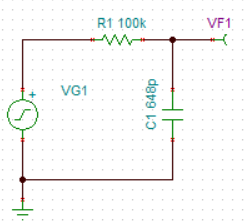thanks

• Well Jian,

You have both a spot noise and integrated noise calculation to make

1. The spot noise is just sqrt(4kT100k) = 40nV/rootHz

2. You have a single pole response which will give an equivalent 1.54*F-3dB noise power bandwidth, then to get Integrated noise take spot noise times eo*sqrt(1.54*2.45kHz) = 2.415uVrms

3. Then,to get noise peak to peak, normally use a 6X crest factor to get 14.7uVpp

All of this covered in this BurrBrown app note,

https://www.ti.com/lit/an/sboa066a/sboa066a.pdf?ts=1616210451757

• Thanks Michael

Next question is why the calculation is not same to the TINA simulation.

TINA simulation result is 1.79uVrms.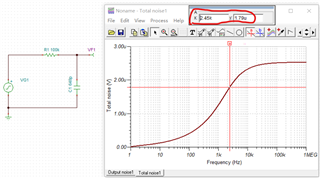• I think I made a mistake earlier, here is the spot noise - 40nV as expected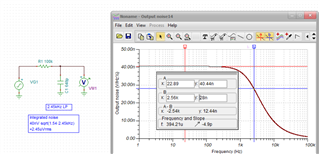Then the integrated noise matches sim,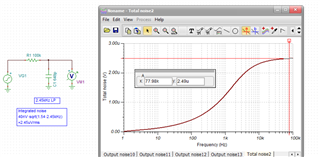• Got it, thanks. so in simulation, the total noise is the maximum value in the plot, not the value at the noise bandwidth, right?

• correct, where it goes flat as the single pole response slowly rolls off and allows little new integrated noise to add in.

• thanks

• HI Michael

After reading the app note, i have some questions on page 7 for equation (12) to (15).

1: Equation 15 including 1/f and broadband noise? or just the 1/f noise?

2: from TI precision lab about the noise calculation,  the 1/f and broadband noise are calculated separately, then using the root sum of the squares.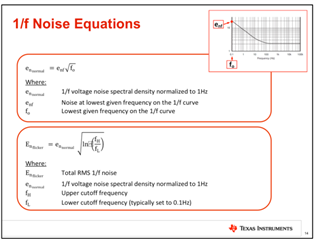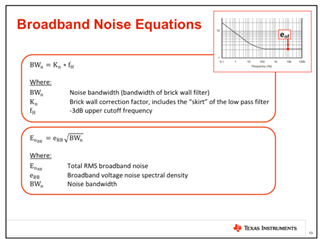here fH is the NPB which is equal to F2 in BB app note.  but how to explain the F3db, F1 corresponding to f0 and fL in TI document??

3: in ADI app note MT-048, the noise is calculated as below: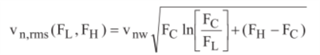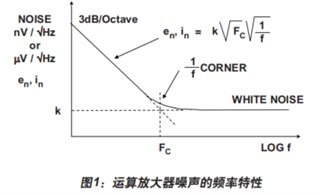Vnw is the noise density. here didn't using the root sum of the squares.

so how to analyze this calculation between ADI and  TI?

thanks!

• Well Jian, I have always known the TI precision and ADI folks showed different results, but I was never interested in trying to correct folks that are not going to accept inputs anyway. But I did finally compare results in the attached word document. The equation I developed many years ago  in a few simple steps exactly matches TINA results.The precision labs equation looks like an approximation and I think the ADI result might just be a typo. HEre is that word file and then the TINA noise macro I was using.

Noise calculations including low frequency region.docx

Voltage noise maco testing.TSC

• thanks very much for your detailed explanation, learned a lot, it is a good way to compare hand calculation with simulation.  BTW, here F-3db  means the 1/f corner frequency,  right?

• You bet,

Yes, here F3dB (without the -sign) means the noise is +3dB up in power at the 1/f corner. Or, the spot noise is 1.414*flatband value

• Got it. thanks, have a nice day!

• There is actually a 2nd mistake in the equation in ADI's MT-048 I had missed - here I discuss this to an ADI guy (who does not care of course)

but I had this open still and noticed that Fc term in the Ln numerator also - should be Fh. And that last Fc term should be FL.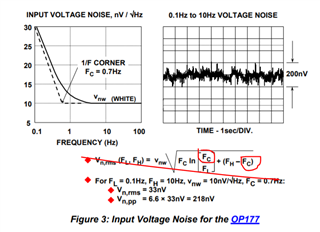If I evaluate using the ADI form above, I get 33nVrms as they show
If I use the corrected equation, I get 36nVrms. And then if I use the more typical 6X crest factor (instead of the 6.6X above) I get 217nVpp - back to their result.
•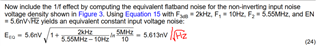Above equation is from app note, Eeq includes 1/f noise and broadband noise, right? the unit is wrong.  The result is 5.613 which is increased a little compared to 5.6, this means the contribution of noise in 1/f region can be negligible, right?

below is another app from Linear, which can validate your thought.  but still has some confusion.

https://www.analog.com/media/en/reference-design-documentation/design-notes/dn015f.pdf

in this document, didn't include the NPB , for single-pole LF, should be 1.57*F-3db, here should be 1.57 * FH = 1.57*20KHz, right?

Thanks & Regards

• Morning Jian,

You are correct that one equation should have the /root(Hz) units.

And yes the single pole conversion from F-3dB to Noise power bandwidth is a 1.57 factor (not the 1.54 I had shown earlier) - equal to pi/2

Thanks for the LTC app note, yes this equation matches what I had developed for that sboa066a 1996 app note,

Well the cut and paste is not working this morning, but that front page equation in that LTC dn015 matches exactly.

• Hi Michael

I did the calculation using the model in LTC app note. please help to review the steps. thanks again!

Noise Calculation.docx

• Morning Jian,

You raise a number of interesting issues in your calculations.

1. You are assuming the noise terms are all self limited by the Gainbandwidth product. Not really, only the non-inverting input terms self limit, for instance the feedback R noise shows up directly at the output while the Rg noise does get limited.

2. Normally I assume something else setting the NPB like a post RC filter that will control the integrated noise for whatever spectrum shows up at the output - in final app, you should not really depend on self limiting to get a repeatable results as the GBP has quite a lot of variation.

3. I normally use that assumed postfilter NPB to 1st calculate the effective spot noise over that span for any term having a 1/f content (current and voltage noise of the op amp, not the resistors).

4. Then I take the RMS of the separate terms to get the total flat spot noise at the output that will integrate to the same noise power as the actual device

5. then finally take that RMS spot times the NPB you assumed at the beginning.

Really interesting that the LT1037 showed a worst case noise, that is more bold than anyone these days would report. However, it is often not the case that the model captures the 1/f information, TINA V11 has a LT1037 model, here is the voltage noise - no 1/f in that model,

Well darn, the cut and paste is not working again this morning. I will continue offline to test and fix this with external noise source if possible -